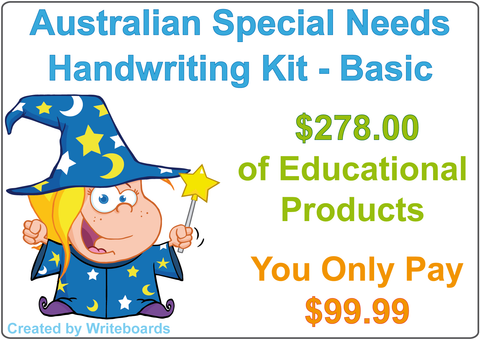Australian Money - Coins

Australian Money - Coin Pack

These eighty worksheets (including flashcards) will teach your child all about coin combinations up to five dollars (\$5.00).

• Australian money posters (pictures of all coins and notes in order to \$100.00).
• Coin combination poster for 10 and 20 cents (x 1), Coin combination poster for 50 cents (x 1), Coin combination posters for \$1.00 (x 2), Coin combination posters for \$2.00 (x 3), Coin combination posters for \$5.00 (x 4).

Worksheets completed on a circle grid:

• Five cents addition (x 3), Ten cents addition (x 3), Twenty cents addition (3), Fifty cents addition (x 3), Fifty cents subtraction (x 1), One dollar subtraction (x 3), One dollar addition (x 3), Two dollars addition (x 3), Two dollars subtraction (x 3).

Worksheets that ask your child to work out what change they will get from the shop:

• Fifty cents (x 1), One dollar (x 3), Two dollars (x 6).

Flashcards:
Five cents, Ten cents, Twenty cents, Fifty cents, One dollar, Two dollars, Five dollars, Ten dollars, Twenty dollars, Fifty dollars and One hundred dollars.

Grids:
Blank 100 grid and Blank 200 grid.